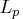Previous Page | Next Page

 Language Reference

## Descriptive Statistics Functions and Subroutines

 CMISS returns the number of missing values for numeric or character matrices CSS returns the corrected sum of squares CV returns the coefficient of variation GEOMEAN returns the geometric mean EUCLID returns the Euclidean norm of the nonmissing arguments GEOMEANZ returns the geometric mean without fuzzing the values of the arguments that are approximately 0 HARMEAN returns the harmonic mean HARMEANZ returns the harmonic mean without fuzzing the values of the arguments that are approximately 0 IQR returns the interquartile range KURTOSIS returns the kurtosis LARGEST returns the kth largest nonmissing value LPNORM returns thenorm of the nonmissing arguments MAX returns the largest value MAD returns the median absolute deviation from the median MEDIAN computes median values MEAN returns the arithmetic mean (average) MIN returns the smallest value N returns the number of nonmissing values NMISS returns the number of missing values ORDINAL returns any specified order statistic PCTL computes percentiles RANGE returns the range of values RMS returns the root mean square SKEWNESS returns the skewness SMALLEST returns the kth smallest nonmissing value SUM returns the sum of the nonmissing arguments STD returns the standard deviation CALL STDIZE standardizes the values of one or more variables STDERR returns the standard error of the mean SUMABS returns the sum of the absolute values of the nonmissing arguments USS returns the uncorrected sum of squares VAR returns the variancePrevious Page | Next Page | Top of Page